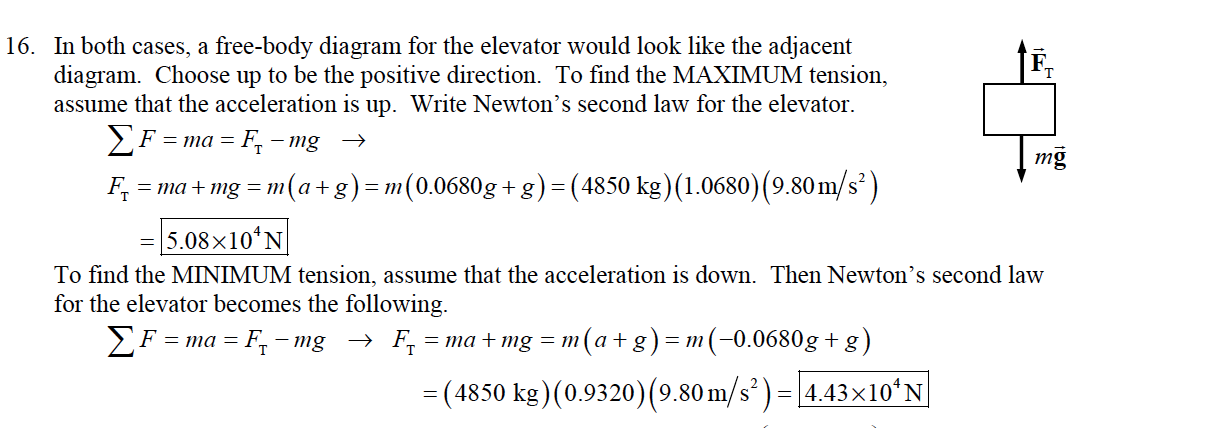# In both cases, a free-body diagram for the elevator would look like the adjacent diagram. Choose up to be the positive direction. To find the MAXIMUM tension, assume that the acceleration is up. Write Newton's second law for the elevator. 16. mg F ="la + mg = m(a+g)-m(0.0680g+g)-(4850 kg)(1 0680)(9.80m/s2) 5.08x10'N To find the MINIMUM tension, assume that the acceleration is down. Then Newton's second law for the elevator becomes the following. - (4850 kg) (0.9320) (9.80m/s2)-4.43x10 N

Question

an elevator (mass 4850kg) is to be designed so that the max acceleration is 0.0680g. what are the max and min forces the motor should exert on the suporting cable?

1. why did they add 1. wouldn't it just be Fn=m(a+g), which would be 4850(0.680g+ 9.8)?help_outlineImage TranscriptioncloseIn both cases, a free-body diagram for the elevator would look like the adjacent diagram. Choose up to be the positive direction. To find the MAXIMUM tension, assume that the acceleration is up. Write Newton's second law for the elevator. 16. mg F ="la + mg = m(a+g)-m(0.0680g+g)-(4850 kg)(1 0680)(9.80m/s2) 5.08x10'N To find the MINIMUM tension, assume that the acceleration is down. Then Newton's second law for the elevator becomes the following. - (4850 kg) (0.9320) (9.80m/s2)-4.43x10 N fullscreen

### Want to see this answer and more?

Experts are waiting 24/7 to provide step-by-step solutions in as fast as 30 minutes!*

*Response times vary by subject and question complexity. Median response time is 34 minutes and may be longer for new subjects.
Tagged in
SciencePhysics

### Newtons Laws of Motion